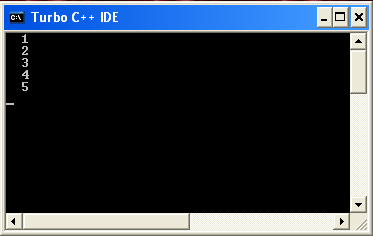# Pass Array In Function

## C++ Program to Pass Array in Function

In below program we pass array in function as an arguments and finally print all elements of an array.

## Example

```
#include<iostream.h>
#include<conio.h>

void pass(int[],int);
void main()
{
int a[]={1,2,3,4,5};
clrscr();
pass(a,5);
getch();
}
void pass(int b[],int n)
{
int i;
for(i=0;i<n;i++)
{
cout<<"/n"<<b[i];
}
}
```

## Output

```1
2
3
4
5
```

Output